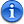Page 1 of 1

### Newton's MethodPosted: February 18th, 2012, 9:38 pm
Newton's Method

This is a method for approximating solutions to equations.The blue line is the tangent line at x[sub]0[/sub]. Where the line crosses the x-axis, it is much closer to the actual solution.If x[sub]n[/sub] is an approximation ofand ifthe next approximation is given by,